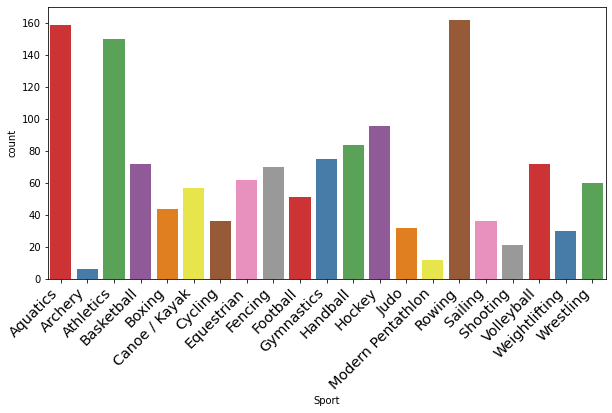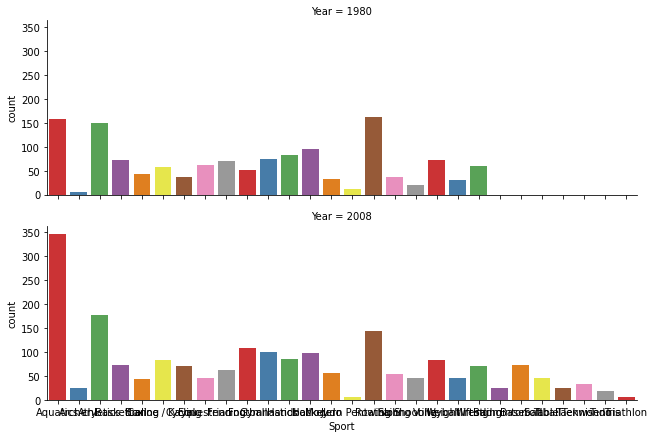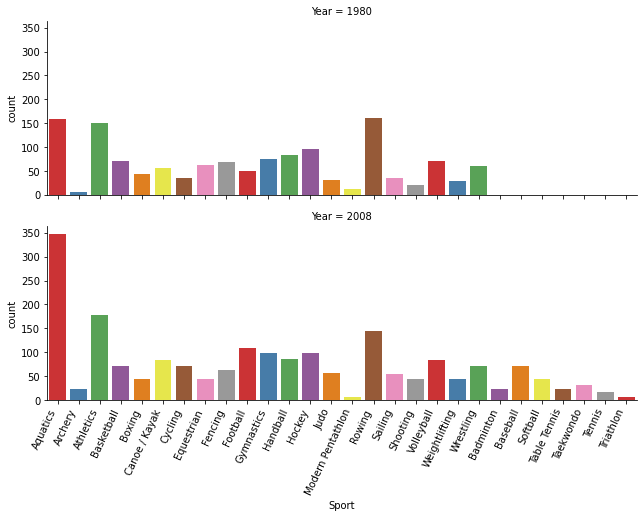There's a common pattern which often occurs when working with charting libraries: drawing charts with all the defaults seems very straightforward, but when we want to change some aspect of the chart things get complicated. This pattern is even more noticable when working with a high-level library like `seaborn` - the library does all sorts of clever things to make our life easier, and lets us draw sophisticated, beautiful charts, so it's frustrating when we want to change something that feels like it should be simple.

Tip: For a full overview of styling charts, check out the chapters on styles and color in the Drawing from Data book.

In this article, we'll take a look at the classic example of this phenomenon - rotating axis tick labels. This seems like such a common thing that it should be easy, but it's one of the most commonly asked questions on StackOverflow for both `seaborn` and `matplotlib`. As an example dataset, we'll look at a table of Olympic medal winners. We can load it into `pandas` directly from a URL:

```import pandas as pd

data
```
City Year Sport ... Medal Country Int Olympic Committee code
0 Athens 1896 Aquatics ... Gold Hungary HUN
1 Athens 1896 Aquatics ... Silver Austria AUT
2 Athens 1896 Aquatics ... Bronze Greece GRE
3 Athens 1896 Aquatics ... Gold Greece GRE
4 Athens 1896 Aquatics ... Silver Greece GRE
... ... ... ... ... ... ... ...
29211 Beijing 2008 Wrestling ... Silver Germany GER
29212 Beijing 2008 Wrestling ... Bronze Lithuania LTU
29213 Beijing 2008 Wrestling ... Bronze Armenia ARM
29214 Beijing 2008 Wrestling ... Gold Cuba CUB
29215 Beijing 2008 Wrestling ... Silver Russia RUS

29216 rows × 12 columns

Each row is a single medal, and we have a bunch of different information like where and when the event took place, the classification of the event, and the name of the athlete that won.

We'll start with something simple; let's grab all the events for the 1980 games and see how many fall into each type of sport:

```import seaborn as sns
import matplotlib.pyplot as plt

# set the figure size
plt.figure(figsize=(10,5))

# draw the chart
chart = sns.countplot(
data=data[data['Year'] == 1980],
x='Sport',
palette='Set1'
)
```Here we have the classic problem with categorical data: we need to display all the labels and because some of them are quite long, they overlap. How are we going to rotate them? The key is to look at what type of object we've created. What is the type of the return value from the `countplot()` function, which we have stored in `chart`?

```type(chart)
```
`matplotlib.axes._subplots.AxesSubplot`

Looks like `chart` is a `matplotlib` `AxesSubplot` object. This actually doesn't help us very much - if we go searching for the documentation for `AxesSubplot` we won't find anything useful. Instead, we have to know that an `AxesSubplot` is a type of `Axes` object, and now we can go look up the documentation for `Axes` in which we find the `set_xticklabels()` method.

Looking at the documentation for `set_xticklabels()` we don't actually see any obvious reference to rotation. The clue we're looking for is in the "Other parameters" section at the end, where it tells us that we can supply a list of keyword arguments that are properties of `Text` objects.

Finally, in the documentation for `Text` objects we can see a list of the properties, including rotation. This was a long journey! but hopefully it will pay off - there are lots of other useful properties here as well. Now we can finally set the rotation:

```plt.figure(figsize=(10,5))
chart = sns.countplot(
data=data[data['Year'] == 1980],
x='Sport',
palette='Set1'
)
chart.set_xticklabels(rotation=45)
```
```---------------------------------------------------------------------------
TypeError                                 Traceback (most recent call last)
<ipython-input-27-059eaf6ffa77> in <module>
5     palette='Set1'
6 )
----> 7 chart.set_xticklabels(rotation=45)

~/.virtualenvs/drawingfromdata/lib/python3.8/site-packages/matplotlib/axes/_base.py in wrapper(self, *args, **kwargs)
61
62         def wrapper(self, *args, **kwargs):
---> 63             return get_method(self)(*args, **kwargs)
64
65         wrapper.__module__ = owner.__module__

~/.virtualenvs/drawingfromdata/lib/python3.8/site-packages/matplotlib/cbook/deprecation.py in wrapper(*args, **kwargs)
449                 "parameter will become keyword-only %(removal)s.",
450                 name=name, obj_type=f"parameter of {func.__name__}()")
--> 451         return func(*args, **kwargs)
452
453     return wrapper

TypeError: _set_ticklabels() missing 1 required positional argument: 'labels'```Disaster! We need to pass `set_xticklabels()` a list of the actual labels we want to use. Since we don't want to change the labels themselves, we can just call `get_xticklabels()`:

```plt.figure(figsize=(10,5))
chart = sns.countplot(
data=data[data['Year'] == 1980],
x='Sport',
palette='Set1'
)
chart.set_xticklabels(chart.get_xticklabels(), rotation=45)

None #don't show the label objects
```This looks better, but notice how the "Modern Pentathlon" label is running into the "Sailing" label? That's because the labels have been rotated about their center - which also makes it hard to see which label belongs to which bar. We should also set the horizontal alignment to "right":

```plt.figure(figsize=(10,5))

chart = sns.countplot(
data=data[data['Year'] == 1980],
x='Sport',
palette='Set1'
)

chart.set_xticklabels(chart.get_xticklabels(), rotation=45, horizontalalignment='right')

None #don't show the label objects
```And just to show a few more things that we can do with `set_xticklabels()` we'll also set the font weight to be a bit lighter, and the font size to be a bit bigger:

```plt.figure(figsize=(10,5))
chart = sns.countplot(
data=data[data['Year'] == 1980],
x='Sport',
palette='Set1'
)

chart.set_xticklabels(
chart.get_xticklabels(),
rotation=45,
horizontalalignment='right',
fontweight='light',
fontsize='x-large'

)

None #don't show the label objects
```In all of these examples, we've been using the object-oriented interface to `matplotlib` - notice that we're calling `set_xticklabels()` directly on the chart object.

Another object is to use the `pyplot` interface. There's a method simply called `xticks()` which we could use like this:

```import matplotlib.pyplot as plt
plt.figure(figsize=(10,5))
chart = sns.countplot(
data=data[data['Year'] == 1980],
x='Sport',
palette='Set1'
)

plt.xticks(
rotation=45,
horizontalalignment='right',
fontweight='light',
fontsize='x-large'
)

None #don't show the label objects
```Notice that when we do it this way the list of labels is optional, so we don't need to call `get_xticklabels()`.

Althought the `pyplot` interface is easier to use in this case, in general I find it clearer to use the object-oriented interface, as it tends to be more explicit.

Everything that we've seen above applies if we're using `matplotlib` directly instead of `seaborn`: once we have an `Axes` object, we can call `set_xticklabels()` on it. Let's do the same thing using `pandas`'s built in plotting function:

```chart = data[data['Year'] == 1980]['Sport'].value_counts().plot(kind='bar')
chart.set_xticklabels(chart.get_xticklabels(), rotation=45, horizontalalignment='right')

None
```## Dealing with multiple plots

Let's try another plot. One of the great features of `seaborn` is that it makes it very easy to draw multiple plots. Let's see how the distribution of medals in each sport changed between 1980 and 2008:

```chart = sns.catplot(
data=data[data['Year'].isin([1980, 2008])],
x='Sport',
kind='count',
palette='Set1',
row='Year',
aspect=3,
height=3
)
```As before, the labels need to be rotated. Let's try the approach that we used before:

```chart = sns.catplot(
data=data[data['Year'].isin([1980, 2008])],
x='Sport',
kind='count',
palette='Set1',
row='Year',
aspect=3,
height=3
)
chart.set_xticklabels(chart.get_xticklabels(), rotation=45, horizontalalignment='right')
```
```---------------------------------------------------------------------------
AttributeError                            Traceback (most recent call last)
<ipython-input-8-69ed9d536d8c> in <module>
8     height=3
9 )
---> 10 chart.set_xticklabels(chart.get_xticklabels(), rotation=45, horizontalalignment='right')

AttributeError: 'FacetGrid' object has no attribute 'get_xticklabels'```We run into an error. Note that the missing attribute is not `set_xticklabels()` but `get_xticklabels()`. The reason why this approach worked for `countplot()` and not for `factorplot()` is that the output from `countplot()` is a single `Axes` object, as we saw above, but the output from `factorplot()` is a `seaborn` `FacetGrid` object:

```type(chart)
```
`seaborn.axisgrid.FacetGrid`

whose job is to store a collection of multiple axes - two in this case. So how to rotate the labels? It turns out that `FacetGrid` has its own version of `set_xticklabels` that will take care of things:

```chart = sns.catplot(
data=data[data['Year'].isin([1980, 2008])],
x='Sport',
kind='count',
palette='Set1',
row='Year',
aspect=3,
height=3
)
chart.set_xticklabels(rotation=65, horizontalalignment='right')

None
```The `pyplot` interface that we saw earlier also works fine:

```chart = sns.catplot(
data=data[data['Year'].isin([1980, 2008])],
x='Sport',
kind='count',
palette='Set1',
row='Year',
aspect=3,
height=3
)
plt.xticks(rotation=65, horizontalalignment='right')

None
```And, of course, everything that we've done here will work for y-axis labels as well - we typically don't need to change their rotation, but we might want to set their other properties. As an example, let's count how many medals were won at each Olypmic games for each country in each year. To keep the dataset managable, we'll just look at countries that have won more than 500 metals in total:

```by_sport = (data
.groupby('Country')
.filter(lambda x : len(x) > 500)
.groupby(['Country', 'Year'])
.size()
.unstack()
)
by_sport
```
Year 1896 1900 1904 ... 2000 2004 2008
Country
Australia 2.0 5.0 NaN ... 183.0 157.0 149.0
Canada NaN 2.0 35.0 ... 31.0 17.0 34.0
China NaN NaN NaN ... 79.0 94.0 184.0
East Germany NaN NaN NaN ... NaN NaN NaN
France 11.0 185.0 NaN ... 66.0 53.0 76.0
... ... ... ... ... ... ... ...
Russia NaN NaN NaN ... 188.0 192.0 143.0
Soviet Union NaN NaN NaN ... NaN NaN NaN
Sweden NaN 1.0 NaN ... 32.0 12.0 7.0
United Kingdom 7.0 78.0 2.0 ... 55.0 57.0 77.0
United States 20.0 55.0 394.0 ... 248.0 264.0 315.0

17 rows × 26 columns

If the use of two `groupby()` method calls is confusing, take a look at this article on grouping. The first one just gives us the rows belonging to countries that have won more than 500 medals; the second one does the aggregation and fills in missing data. The natural way to display a table like this is as a heatmap:

```plt.figure(figsize=(10,10))
g = sns.heatmap(
by_sport,
square=True, # make cells square
cbar_kws={'fraction' : 0.01}, # shrink colour bar
cmap='OrRd', # use orange/red colour map
linewidth=1 # space between cells
)
```This example is perfectly readable, but by way of an example we'll rotate both the x and y axis labels:

```plt.figure(figsize=(10,10))
g = sns.heatmap(
by_sport,
square=True,
cbar_kws={'fraction' : 0.01},
cmap='OrRd',
linewidth=1
)

g.set_xticklabels(g.get_xticklabels(), rotation=45, horizontalalignment='right')
g.set_yticklabels(g.get_yticklabels(), rotation=45, horizontalalignment='right')
None # prevent the list of label objects showing up annoyingly in the output
```OK, I think that covers it. That was an agonizingly long article to read just about rotating labels, but hopefully it's given you an insight into what's going on. It all comes down to understanding what type of object you're working with - an `Axes`, a `FacetGrid`, or a `PairGrid`.

If you encounter a situation where none of these work, drop me an email at martin@drawingwithdata.com and I'll update this article!